# Subtraction

1
One term is 1
2
One term is 2
3
One term is 3
4
One term is 4
5
One term is 5
6
One term is 6
7
One term is 7
8
One term is 8
9
One term is 9
10
One term is 10
11
One term is 11
12
One term is 12
13
One term is 13
14
One term is 14
15
One term is 15
16
One term is 16
17
One term is 17
18
One term is 18
19
One term is 19
20
One term is 20
21
One term is 21-25
22
One term is 26-30
23
One term is 31-35
24
One term is 36-40
25
One term is 41-45
Difficult
26
One term is 46-50
27
One term is 51-55
28
One term is 56-60
29
One term is 61-65
30
One term is 66-70
31
One term is 71-75
32
One term is 76-80
33
One term is 81-85
34
One term is 86-90
35
One term is 91-100
Do you want to play locked game level?

## Recent activities in this game

Someone had a problem at level 13. One term is 13 .
21 hours ago after 49 seconds of playing.
Someone had a problem at level 13. One term is 13 .
21 hours ago after 15 seconds of playing.
Someone got all questions correct at level 6. One term is 6 .
21 hours ago after 22 seconds of playing.
Someone got all questions correct at level 1. One term is 1 .
21 hours ago after 6 seconds of playing.
Someone got all questions correct at level 1. One term is 1 .
21 hours ago after 9 seconds of playing.
Someone got all questions correct at level 1. One term is 1 .
2022-05-27 00:08 after 9 seconds of playing.
Someone got all questions correct at level 14. One term is 14 .
2022-05-25 23:33 after 20 seconds of playing.
Someone got all questions correct at level 1. One term is 1 .
2022-05-25 02:38 after 39 seconds of playing.
Someone got all questions correct at level 6. One term is 6 .
2022-05-24 20:05 after 16 seconds of playing.
Someone got all questions correct at level 6. One term is 6 .
2022-05-24 18:19 after 19 seconds of playing.

Practice subtraction or minus with a skill training math game. Can you subtract and find the difference between two numbers?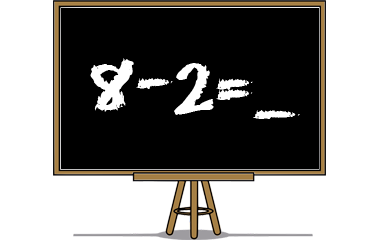## Game instructions for subtraction

Choose a number from the buttons above. This will give you up to ten tasks where you have to find the difference between the two terms. Can you solve all the subtractions in a level, you will get one knowledge points and the button for that level turns green.

### Subtraction in the language of mathematics

Subtraction belongs to the four basic rules of arithmetic and the correct way of talking about it to subtract.

A term subtracted from another term forms a difference:
 7 - 5 = 2 term - term = difference

### Calculation of knowledge points

Each completed game level gives 1 knowledge point in Subtraction. The maximum number of points (35 knowledge points) is achieved when you pass all 35 levels. You'll get a bronze medal when you complete a level 2 times and a silver medal after 5 completed rounds. A gold medal will be received after 10 completed rounds. In Subtraction, the maximum number of collectable gold medals is 35. You only earn knowledge points for levels that has not been cleared before. A cleared level has a green background above. Even when you have completed a level, you can continue practicing at that level, but it gives you no more knowledge points.

### Similar games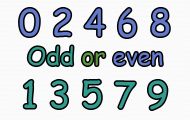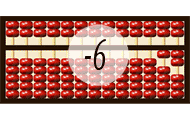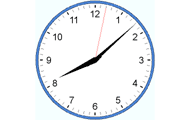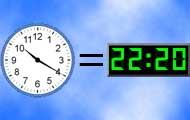Math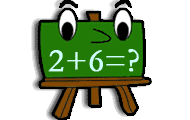MathMathMath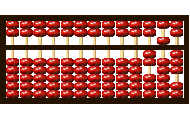MathMath

Subject
Math
Target group
Practices
Numbers Subtraction Mental calculation
Game creator
Updated
2019-10-02
Published online
2011-11-11
Unique id
5
Saved by
5-10 users
Operating system
Browser
Price
Free of charge

3.9
Total 344
5
216
4
28
3
19
2
20
1
61

### Report

If you find a spelling mistake or something else that should be corrected, feel free to contact the game creator or us at Helpful Games.

Write a comment below to contact the game creator or

There are a total of 4 comments on this knowledge game.

A
27 August 2020 16:48
This game is so awesome you can use these4 your kids this game is legit Oakland A's game every day and that's awesome.
O
25 March 2020 13:03
I love this game.omg is so fun
L
23 October 2018 21:14
This game is perfect to learn subtraktion.
G
10 November 2014 14:13
This game is perfect to learn subtraction. An almost perfect game!
Weekly top list
1.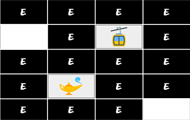Brain training
2.Brain training
3.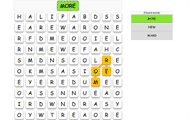English
4.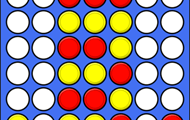Brain training
5.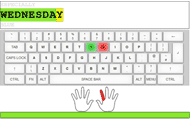Computer and media

More plus membership benefits